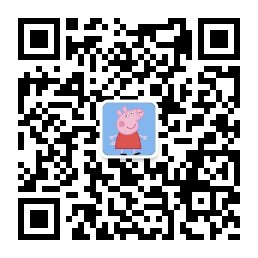# Rust学习之所有权

2020/01/14 共 7164 字，约 21 分钟# 什么是所有权

Rust的主要特征是所有权。尽管该功能易于解释，但对其余语言有深远的影响。

## 所有权规则

• Rust中的每个值都有一个变量，称为其所有者。
• 一次只能有一个所有者。
• 当所有者超出范围时，该值将被删除。

## 变量范围

``````//后面切面中会讲到：字符串文字类型是＆str，这是不可变的引用，它是指向二进制文件的特定点的切片。这也是字符串文字（常量）不可变的原因。
let s = "hello";
``````

``````{                      // s 无效，还没有被定义
let s = "hello";   // 从现在开始，s是有效的

// 用s处理一些事情
}  // 此作用域已经结束，并且s不再有效
``````

• 当s进入范围时，它是有效的。
• 它保持有效，直到超出范围。

## String 类型

``````let s = String::from("hello");
``````

``````let mut s = String::from("hello");

s.push_str(", world!"); // push_str() 将文字附加到字符串，必须使用mut，否则s不可变！

println!("{}", s); //打印 hello, world!
``````

## 内存和分配

• 必须在运行时从操作系统请求内存。
• 在完成String操作后，我们需要一种将该内存返回给操作系统的方法。

Rust采取了另一条路径：拥有内存的变量超出范围后，内存将自动返回。这是范围示例的一个版本，其中使用String而不是字符串常量：

``````{
let s = String::from("hello"); // 从现在开始，s是有效的

// 使用s做一些事情
}    // 此范围现已结束，s不再有效
``````

## 变量与数据交互的方式：移动（move）

``````let x = 5;
let y = x;
``````

``````let s1 = String::from("hello");
let s2 = s1;
````````````let s1 = String::from("hello");
let s2 = s1;

println!("{}, world!", s1);
``````

``````error[E0382]: use of moved value: `s1`
--> src/main.rs:5:28
|
3 |     let s2 = s1;
|         -- value moved here
4 |
5 |     println!("{}, world!", s1);
|                            ^^ value used here after move
|
= note: move occurs because `s1` has type `std::string::String`, which does
not implement the `Copy` trait
``````## 变量与数据交互的方式：克隆（clone）

``````let s1 = String::from("hello");
let s2 = s1.clone();

println!("s1 = {}, s2 = {}", s1, s2);
``````

## 仅堆栈数据：复制（copy）

``````let x = 5;
let y = x;

println!("x = {}, y = {}", x, y);
``````

Rust具有一个特殊的注解，称为Copy特质，我们可以将其放置在存储在堆栈中的整数等类型上。如果类型具有Copy特质，则分配后仍然可以使用较旧的变量。如果该类型或其任何部分实现了Drop特性，Rust将不允许我们使用Copy特质来注解该类型。如果在值超出范围时该类型需要特殊处理，并向该类型添加Copy注解，则会出现编译时错误。要了解如何将Copy注解添加到您的类型中，请参阅“可导出特质”。

• 所有整数类型，例如u32。
• 布尔类型bool，值为true和false。
• 所有浮点类型，例如f64。
• 字符类型，例如char。
• 元组（如果它们仅包含Copy的类型）。例如，（i32，i32）则是Copy，但（i32，String）不是。

## 所有权和函数

``````fn main() {
let s = String::from("hello");  // s进入范围

takes_ownership(s);             // s的值移动到函数，所以在这里不再有效

let x = 5;                      // x进入范围

makes_copy(x);                  // x将移动到函数
// 但是i32是Copy，所以之后还可以使用

}

fn takes_ownership(some_string: String) {
println!("{}", some_string);
} //在这里，some_string超出范围并调用`drop`。内存释放

fn makes_copy(some_integer: i32) {
println!("{}", some_integer);
} //在这里，some_integer超出范围。没什么特别的发生。
``````

## 返回值和范围

``````fn main() {
let s1 = gives_ownership();         // lets_ownership移动其返回值到s1中

let s2 = String::from("hello");     // s2进入范围

let s3 = takes_and_gives_back(s2);  // s2被移入takes_and_gives_back（s2此后不可用）,  takes_and_gives_back的返回值被移动到s3
} // 此处，s3超出范围并被丢弃。 s2超出范围，但是移动了，所以什么也没发生，s1超出范围并被丢弃.

fn gives_ownership() -> String {             // gives_ownership会其返回值移动到调用它的函数中

let some_string = String::from("hello"); // some_string进入范围

some_string                              // 返回some_string字符串并移到调用函数

}

// take_and_gives_back将获取一个String并返回一个
fn takes_and_gives_back(a_string: String) -> String { // a_string进入范围

a_string  // 返回a_string并移至调用函数
}
``````

``````fn main() {
let s1 = String::from("hello");

let (s2, len) = calculate_length(s1);

println!("The length of '{}' is {}.", s2, len);
}

fn calculate_length(s: String) -> (String, usize) {
let length = s.len(); // len() 返回字符串的长度

(s, length)
}
``````